# C Program to Show a Man Walking in Rain

• Last Updated : 13 Apr, 2022

In Turbo C graphics the graphics.h functions are used to draw different shapes(like a circle, rectangle, etc), and display text(any message) in different formats (different fonts and colors). By using graphics.h programs, animations, and also games can be designed. These can be useful for beginners.

This program demonstrates how a man walks in the rain with an umbrella, and when a key is pressed, the rain ceases, and a rainbow appears in the sky.

The header files stdio.h, conio.h, and graphics.h are included because functions defined in these files are used in the program. The #define command is used to define some important elements used throughout the program.

Functions Used:

• getmaxx(): The graphics.h header file contains the getmaxx() function that returns the maximum X coordinate for the current graphics mode and driver.
• getmaxy(): The function getmaxy() returns the maximum Y coordinate for the current graphics mode and driver.
• setcolor(): The setcolor() function sets the current drawing color to the new color.
• rectangle(): rectangle() draws a rectangle. To draw the rectangle, you will need the coordinates of the left top corner and the right bottom corner. The left coordinate specifies the X-coordinate of the top left corner, the top coordinate specifies the Y-coordinate of the top left corner, the right coordinate specifies the X-coordinate of the right bottom corner, and the bottom coordinate specifies the Y-coordinate of the right bottom corner.
• line(): The line() function draws a line from a point (x1,y1) to a point (x2,y2), i.e. (x1,y1) and (x2,y2) are the endpoints of the line.
• setfillstyle() and floodfill(): In graphics.h, setfillstyle() sets the current fill pattern and fill color. floodfill() fills an enclosed area.
• circle(): The graphics. h header file contains the function circle() that draws a circle with a center at (x, y) and a given radius.
• pieslice(): This function draws and fills a pie slice with a given radius r and a center at (x, y). The slice starts from and ends at s_angle and e_angle, respectively.
• rand(): rand() function is used in C to generate random numbers. If we generate a sequence of random numbers. Each time the program runs, the rand() function will create the same sequence again and again.
• delay(): The delay function suspends the execution of a program for a specified period of time.
• arc(): The header file graphics.h contains the arc() function which draws an arc with a center at (x, y) and a given radius.
• getch(): The getch() function holds the output of the program.
• kbhit(): Determines whether a key has been pressed. Include the header file “conio. h” in your program to use it.

Custom Functions:

• hut(): Creates a hut.
• DrawManAndUmbrella(): Creates a man holding an umbrella.
• Rain(): Creates the rainfall.
• rainbow(): Creates a rainbow.

Approach:

In this program, we will create scenery with a hut, sun, and rainfall. In this scenery, a man holds an umbrella and walks through the ground. When a key is pressed, the rain stops, and a rainbow appears.

• Ground Level: To create a ground line, we will first use GroundY ScreenHeight to define the ground level.
• Hut: The hut will be built for the scenery. For the base and roof, rectangles and lines will be used. The hut will be colorful.
• Man and Umbrella: Build a man holding an umbrella. A circle forms the head of the man, and lines make up his body. Lines representing the legs have variable coordinates so it appears as if the man is walking on the ground. Using the pieslice function we will create the body of an umbrella and lines for the stick.
• Rain: Create rain using the rand() function to generate random pixels and create small lines to create a rain effect
• Circle: To create a sun in the top left corner we will use a circle.
• Rainbow: Create a rainbow in the top left corner using the arc function. The delay function gives it an animation.
• Rainfall continues until any key is pressed.
• The rainbow appears when a key is pressed.

Below is the C program to implement the above approach:

## C

 `// C program to implement``// the above approach``#include ``#include ``#include ``#define ScreenWidth getmaxx()``#define ScreenHeight getmaxy()``#define GroundY ScreenHeight * 0.75``int` `ldisp = 0;`` ` `// Creating a hut``void` `hut()``{``    ``setcolor(WHITE);``    ``rectangle(150, 180, 250, 300);``    ``rectangle(250, 180, 420, 300);``    ``rectangle(180, 250, 220, 300);`` ` `    ``line(200, 100, 150, 180);``    ``line(200, 100, 250, 180);``    ``line(200, 100, 370, 100);``    ``line(370, 100, 420, 180);`` ` `    ``setfillstyle(SOLID_FILL, BROWN);``    ``floodfill(152, 182, WHITE);``    ``floodfill(252, 182, WHITE);``    ``setfillstyle(SLASH_FILL, BLUE);``    ``floodfill(182, 252, WHITE);``    ``setfillstyle(HATCH_FILL, GREEN);``    ``floodfill(200, 105, WHITE);``    ``floodfill(210, 105, WHITE);``}`` ` `// Drawing a Man with ``// an umbrella``void` `DrawManAndUmbrella(``int` `x, ``                        ``int` `ldisp)``{``    ``circle(x, GroundY - 90, 10);``    ``line(x, GroundY - 80, x, ``         ``GroundY - 30);``    ``line(x, GroundY - 70, ``         ``x + 10, GroundY - 60);``    ``line(x, GroundY - 65, x + 10, ``         ``GroundY - 55);``    ``line(x + 10, GroundY - 60, ``         ``x + 20, GroundY - 70);``    ``line(x + 10, GroundY - 55,``         ``x + 20, GroundY - 70);`` ` `    ``line(x, GroundY - 30, ``         ``x + ldisp, GroundY);``    ``line(x, GroundY - 30, ``         ``x - ldisp, GroundY);`` ` `    ``pieslice(x + 20, GroundY - 120, ``             ``0, 180, 40);``    ``line(x + 20, GroundY - 120, ``         ``x + 20, GroundY - 70);``}`` ` `// Creating the Rainfall``void` `Rain(``int` `x)``{``    ``int` `i, rx, ry;``    ``for` `(i = 0; i < 400; i++) ``    ``{``        ``rx = ``rand``() % ScreenWidth;``        ``ry = ``rand``() % ScreenHeight;``        ``if` `(ry < GroundY - 4) ``        ``{``            ``if` `(ry < GroundY - 120 || ``               ``(ry > GroundY - 120 && ``               ``(rx < x - 20 || ``                ``rx > x + 60)))``                ``line(rx, ry, ``                     ``rx + 0.5, ry + 4);``        ``}``    ``}``}`` ` `// Creating the rainbow``void` `rainbow()``{``    ``int` `x, y, i;`` ` `    ``circle(ScreenWidth - 100, ``           ``50, 30);``    ``setfillstyle(SOLID_FILL, ``                 ``YELLOW);``    ``floodfill(ScreenWidth - 100,``              ``50, WHITE);`` ` `    ``ldisp = (ldisp + 2) % 20;``    ``DrawManAndUmbrella(x, ldisp);``    ``hut();``    ``x = getmaxx() / 5;``    ``y = getmaxy() / 5;`` ` `    ``for` `(i = 30; i < 100; i++) ``    ``{``        ``// for animation``        ``delay(50);`` ` `        ``setcolor(i / 10);`` ` `        ``arc(x, y, 0, 180, i - 10);``    ``}``    ``getch();``}`` ` `// Driver code``void` `main()``{``    ``int` `gd = DETECT, gm, x = 0;`` ` `    ``initgraph(&gd, &gm, ``              ``"C:\\TurboC3\\BGI"``);`` ` `    ``// executes till any key ``    ``// is pressed``    ``while` `(!kbhit()) ``    ``{``        ``hut();``        ``circle(ScreenWidth - 100, ``               ``50, 30);``        ``setfillstyle(SOLID_FILL, ``                     ``YELLOW);``        ``floodfill(ScreenWidth - 100, ``                  ``50, WHITE);``        ``line(0, GroundY, ScreenWidth, ``             ``GroundY);``        ``Rain(x);`` ` `        ``ldisp = (ldisp + 2) % 20;``        ``DrawManAndUmbrella(x, ldisp);``        ``delay(20);``        ``cleardevice();``        ``x = (x + 2) % ScreenWidth;``    ``}``    ``// if the key is pressed the ``    ``// rain stops, rainbow appears``    ``ldisp = (ldisp + 2) % 20;``    ``DrawManAndUmbrella(x, ldisp);``    ``rainbow();``    ``getch();``}`

Steps to Run this Program:

Step 1: Go to C:\TURBOC3\BIN and move the scenery.c file here.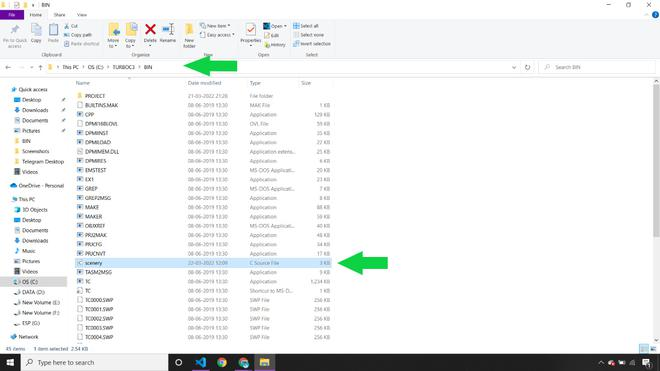Moving scenery.c file in C:\TURBOC3\BIN

Step 2: Open Turbo C++.

Step 3: Click on Files and Open Files. You will see the scenery.c file in the list.

Step 4: Select the file and press open to open scenery.c file in Turbo C++.
Opening scenery.c file in turbo c++

Step 5:  Click on Run to run the graphics program.

Output: Observe a man holding an umbrella walking in the rain with a hut and a sun in the scenery.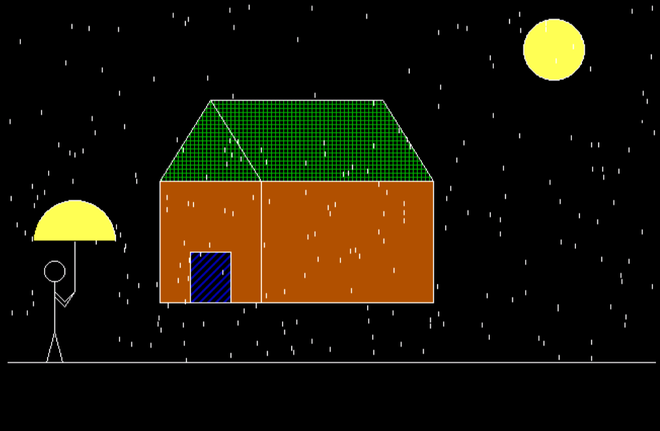Man walking in the rain in C graphics

When a key is pressed, the rain stops, and a rainbow appears.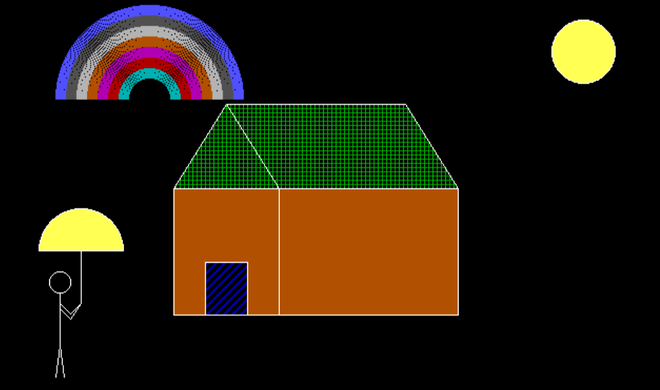The rainbow appears on the keypress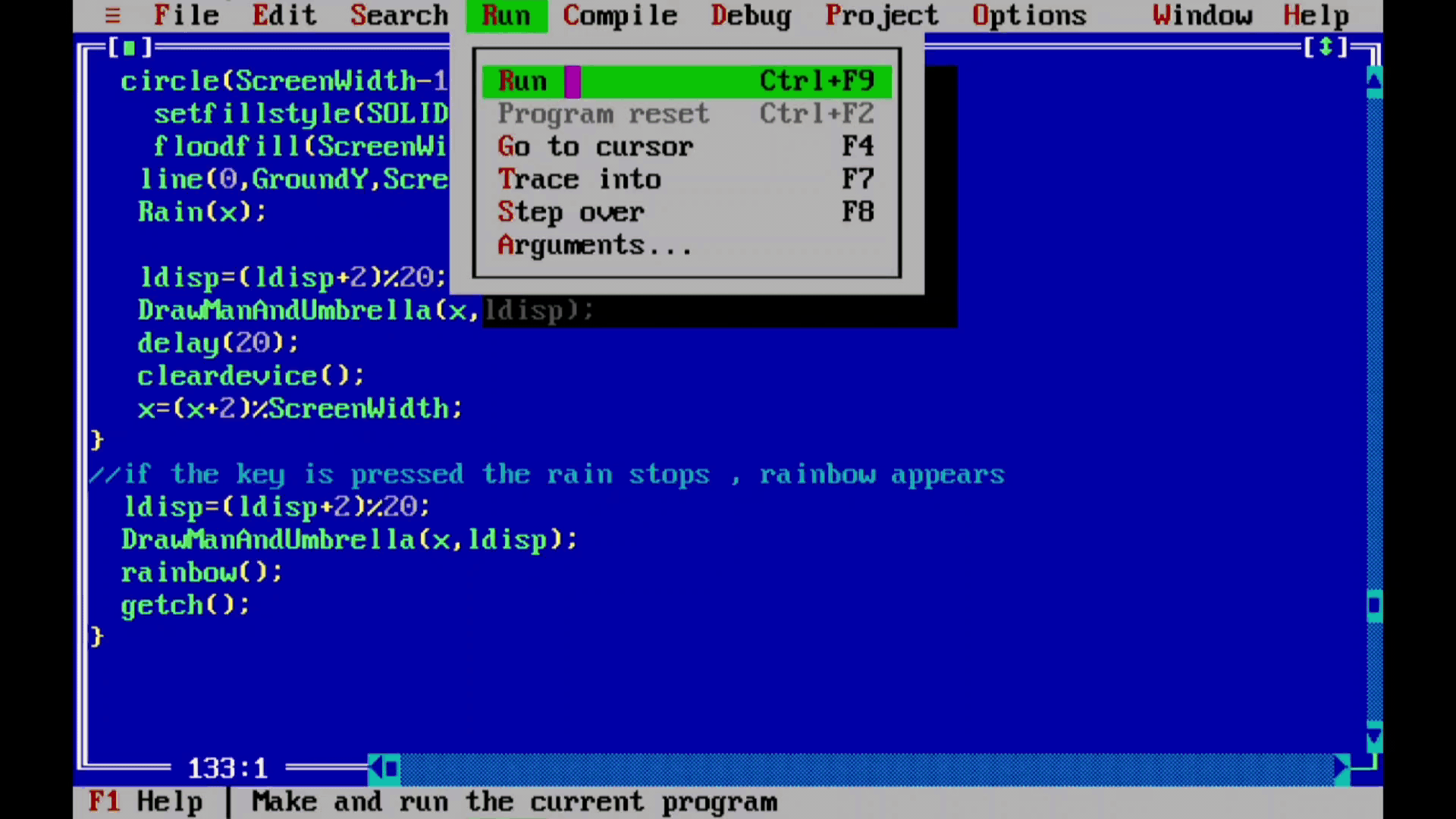Working Output

My Personal Notes arrow_drop_up